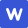2022年高考真题 理科数学 (全国乙卷)

|

# 理科数学 热门试卷

X 查看更多试卷

1、单选题
1
2
3
4
5
6
7
8
9
10
11
12
2、填空题
13
14
15
16
3、简答题
17
18
19
20
21
22
23
• 真题试卷
• 模拟试卷
• 预测试卷

1

|

A2∈M

B3∈M

C4∉M

D5∉M

A
1

|

Aa=1,b=-2

Ba=-1,b=2

Ca=1,b=2

Da=-1,b=-2

A
1

|

A-2

B-1

C1

D2

C
1

|

A<

B<

C<

D<

D
1

|

A2

B2

C3

D3

B
1

|

A3

B4

C5

D6

B
1

|

A平面 EF⊥平面

B平面 EF⊥平面 BD

C平面 EF∥平面 AC

D平面 EF∥平面 D

A
1

|

A14

B12

C6

D3

D
1

|

A

B

C

D

### 正确答案

C
1

|

Ap与该棋手和甲、乙、丙的比赛次序无关

B该棋手在第二盘与甲比赛，p最大

C该棋手在第二盘与乙比赛，p最大

D该棋手在第二盘与丙比赛，p最大

D
1

|

A

B

C

D

C
1

|

A-21

B

C-23

D-24

D

1

|

1

|

1

|

3

1

|

### 正确答案

1

|

(2)若a=5，cosA=，求△ABC的周长.

### 正确答案

(1)在△ABC中,sinC=sin(A+B),sinB=sin(C+A),因为

sin(A+B)sin(A-B)=sin(C+A)sin(C-A),所以

-=-,2=+,2=+.

(2)   由+=50,cosA=,*2bc=50-25,2bc=31,bc=,=++2ab=81,b+c=9,△ABC的周长为9+5=14

1

|

（1）证明:平面BED⊥平面ACD;

（2）设AB=BD=2，ACB=60°，点F在BD上，当△AFC 的面积最小时，求CF与平面ABD所成的角的正弦值.

### 正确答案

(2)由（1）知，设E为原点，以直线EA,EB,ED分别为x,y,z轴建立空间直角坐标系.AB=BD=2,等腰△ABC中ACB=60°，则△ABC为等边三角形，所以BE=,DE=1,AE=EC=1,设A(1,1,0)B(0,0)C(-1,0,0)D(0,0,1),设⊥平面ABD,(x,y,z),则*=0,*=0,x=y,x=z,所以=（,1,）,由于△AFC面积最小，EF⊥BD,EF==,DF=,DF=DB,设=(0,,-1),=(0,,-1),=,+-=(1,),设CF与平面ABD所成角为a,则sina=|cos*|==,CF与平面ABD所成的角的正弦值为.

1

|

（1）估计该林区这种树木平均一棵的根部横截面积与平均一棵的材积量;

（2）求该林区这种树木的根部横截面积与材积量的样本相关系数（精确到0.01）；

（3）现测量了该林区所有这种树木的根部横截面积，并得到所有这种树木的根部横截面积总和为186m².已知树木的材积量与其根部横截面积近似成正比.利用以上数据给出该林区这种树木的总材积量的估计值.

### 正确答案

(1)0.6*=0.06m²,3.9*=0.39,该林区这种树木平均一棵的根部横截面积为0.06m²，平均一棵的材积量0.39

(2)r===≈0.97

(3)=kx,0.39=0.06k,k==6.5,=6.5*186=1209,该林区这种树木的总材积量为1209.

1

|

（1）求E的方程;

（2）设过点P（1,-2）的直线交E于M，N两点，过M且平行于x轴的直线与线段AB交于点T，点H满足=．证明:直线HN过定点．

### 正确答案

(1)设椭圆方程为m+n=1(m>0,n>0,m≠n),带入A(0,-2)B(,-1),,得,椭圆方程为+=1

(2)（0，-2）

1

|

（1）当a=1时，求曲线y=在点（0,）处的切线方程;

（2）若在区间（-1,0）,（0，+∞）各恰有一个零点，求a的取值范围

### 正确答案

(1)定义值为=（1，+∞）,=++)=,=ln（1+0）+=0,==2,曲线y=在点（0,）处的切线方程y=(x-0)=2x

(2)当aln（1+0）+=0,不合题意.所以a，令=1+,x[-1，+∞）,'==(x-1-)(x-1+),R,,a,=1,=1+,=1+a,=,当-1（0,1],=1-a0,1,1-=1+(1-xy)10,,,',在[0，+∞）上单调增，=0矛盾，所以a

1

|

（1）写出l的直角坐标方程;

（2）若l与C有公共点，求m的取值范围.

### 正确答案

（1）ρsin(Θ+)+m=0,ρsin(sinΘ+cosΘ)+m=0,即ρsinΘ+ρcosΘ+m=0,x+y+2m

（2）

1

|

（1）abc≤;

（2）++.

### 正确答案

(1)++=13=3,3≤1,abc≤

（2）++,++++=++,b+c2,,,,++